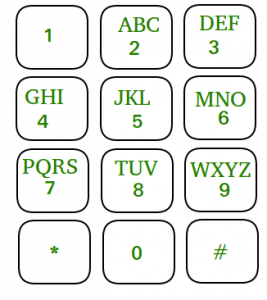Open In App

# Amazon Interview | Set 119 (On-Campus for Internship)

The selection procedure consisted of an online round followed by two Personal Interviews.

Online Round:
The first round had 20 mcq consisting of 3-4 questions from aptitude, 2 questions from os and rest were from Basic C and Data Structure. There were 2 coding questions as well.

Question 1: You are given a phone keypad like following diagram, where each character corresponds to a digit mentioned in the same box.You are given n strings and you have to find their decimal representation. You have to print the string and corresponding decimal representation in descending order.
Like if you are given “amazon” then its corresponding decimal notation will be 262966.
If more than one strings have same decimal notation then you have to print them in the order in which input is given.
(Given that given string consists of lower case alphabets only.)

```Test Case 1:
5
Amazon
Microsoft
Aa
Bb

Output:
642767638  microsoft
262966  amazon
22 aa
22 bb```
```Input: abc
Output:
A
Ab
Ac
Abc
b
Bc
c```

Candidate who could solve at least one question out of 2 coding questions was selected for personal Interviews.

Round 2(P.I. 1) :-
Firstly interviewer had a deep discussion of my project. He also asked me to write code for one of my mini project (Backtracking).
Then he asked two back to back coding questions –

Then he asked the language in which I am comfortable for coding. I said C . Then he asked me to allocate the dynamic memory of 2-d array in C( I used malloc function for this). Then he asked me the difference b/w malloc and new (c++) function.

Round 2 (P.I. 2):-
Firstly he asked me to tell about my academic background. Then he directly put a coding question in front of me.
1. You are given a binary tree and two nodes. You have to print the horizontal distance between them.

Let us say :
Given nodes are B and C : Answer would be 2.
Given nodes are A and E : Answer would be 0.
Given nodes are D and G : Answer would be 4.

We had an long discussion of 45 mins (approx.) on this problem.

One of my friend was asked the following questions:
2. Given a linked list. You have to determine whether or not it is a palindrome in order O(n) time without using auxiliary space.
3. You are given an array of integers . You have to find the maximum sum sequence.(It needs not be contiguous).

After all these rounds I and my friend both were selected 🙂 :). A total of 16 candidates were selected.
I really thank to GEEKSFORGEEKS for all the support and help in my preparation.

If you like GeeksforGeeks and would like to contribute, you can also write an article and mail your article to contribute@geeksforgeeks.org. See your article appearing on the GeeksforGeeks main page and help other Geeks.